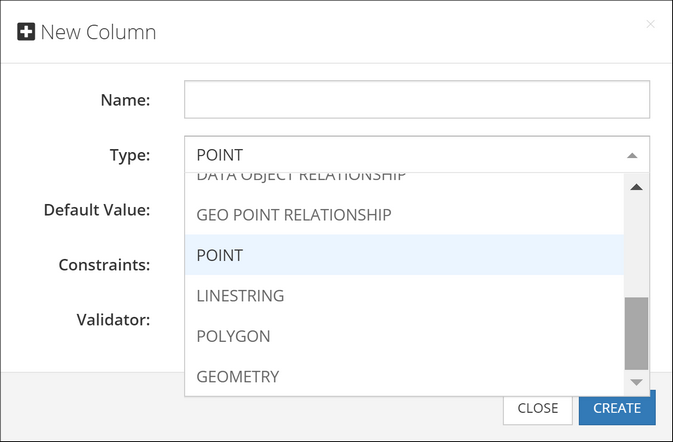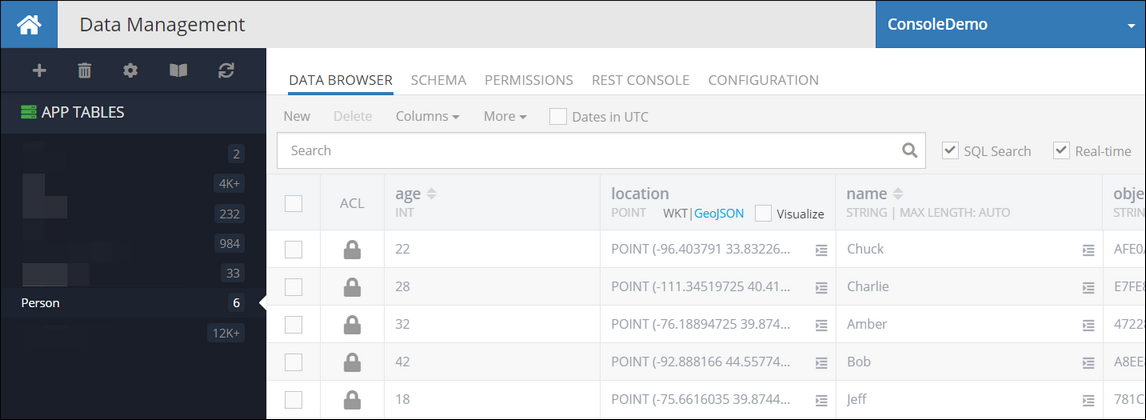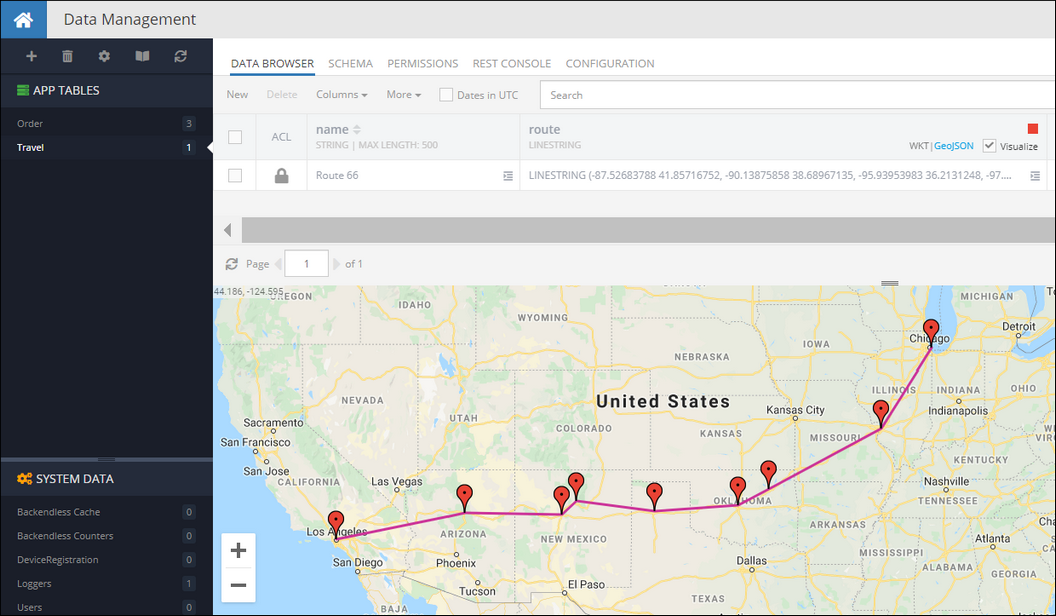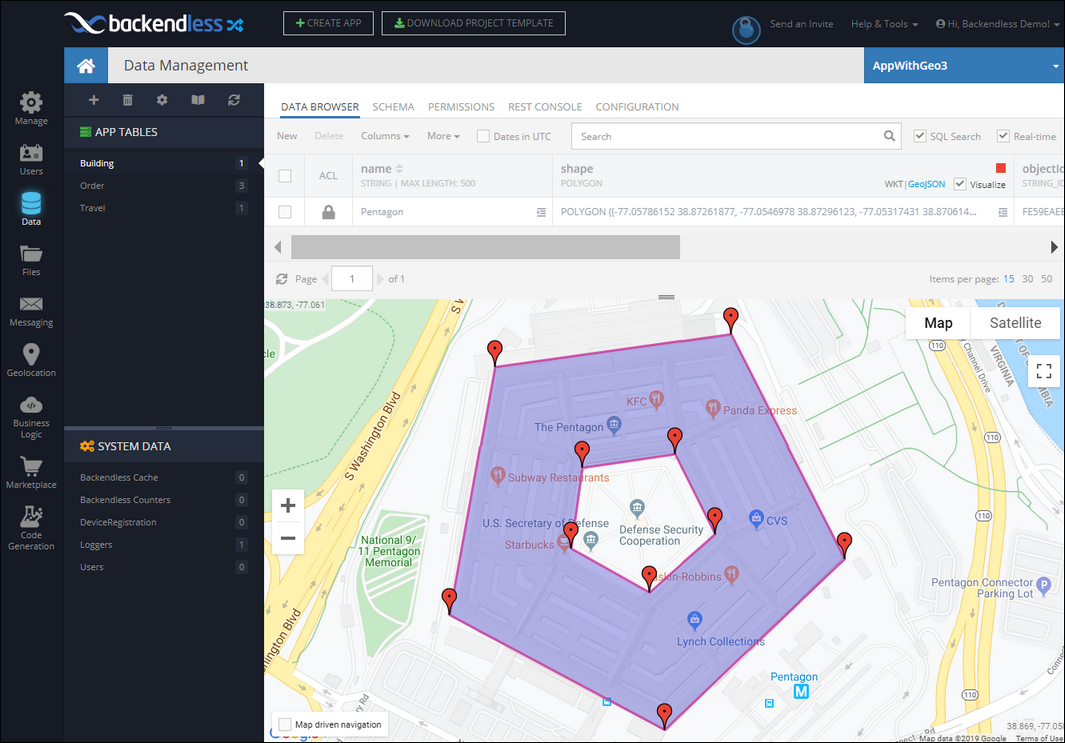# Spatial Data Types¶

Backendless database supports the following spatial data types:

• `POINT` - represents a single point/location in coordinate space. For the points representing locations on a map, the coordinates are longitude and latitude.
• `LINESTRING` - represents a geometry consisting of a collection of points with linear interpolation between them. For example, a linestring can represent a delivery route.
• `POLYGON` - a closed geometrical shape consisting of a single exterior boundary and zero or more interior boundaries, also referred to as holes.

Additionally, Backendless supports a "parent" data type called `GEOMETRY`. This is the base type which can accommodate any of the data types listed above.Spatial data in Backendless can be represented either in the WKT (Well-Known Text) or the GeoJSON formats. Backendless console supports both of these formats for entering new data or updating existing spatial values. Additionally, Backendless SDKs provide built-in classes which make it easier to work with the spatial data for all database operations, including creating, retrieving, updating and deleting spatial data.

## POINT¶

The `POINT` type is used to represent a single point identified by two coordinates in a coordinates space. The coordinates are named X and Y, however, Backendless also handles these coordinates as longitude (X) and latitude (Y) to represent locations on a map. The WKT representation of this type is:

```POINT (longitude latitude)
```

or

```POINT (x y)
```

for example, the `POINT` below points to Dallas, TX

```POINT (-96.7553535 32.8656106)
```

The GeoJSON format for the `POINT` values is:

```{
"type": "Point",
"coordinates": [
longitude or X,
latitude or Y
]
}
```

A `POINT` value stored in the database is represented by the `BLPoint` class in the client application. The class provides access to the point coordinates (`x` and `y`) which can also represent longitude and latitude in cases when the point identifies a location on a map. The class has the constructor and methods listed below. Notice that all `set` methods return the current `Point` object, which allows for convenient "chaining" for property assignments: `pointInstance.setX( value ).setY( value )`:

```// creates a new instance of Point
- (nonnull instancetype)initWithX:(double)x y:(double)y;
- (nonnull instancetype)initWithLongitude:(double)longitude latitude:(double)latitude;

// creates a Point object from a WKT definition
+ (BLPoint * _Nullable)fromWkt:(NSString * _Nonnull)wkt;

// creates a Point object from a GeoJSON definition
+ (BLPoint * _Nullable)fromGeoJson:(NSString * _Nonnull)geoJson;

// Objective-C approach is to use instance properties directly without get/set methods:

// retrieves the x coordinate of the point (same as longitude)
point.x;

// retrieves the y coordinate of the point (same as latitude)
point.y;

// retrieves the longitude coordinate of the point (same as x)
point.longitude;

// retrieves the latitude coordinate of the point (same as y)
point.latitude;

// sets the x coordinate of the point (same as longitude)
point.x = ...;

// sets the y coordinate of the point (same as latitude)
point.y = ...;

// sets the longitude coordinate of the point (same as x)
point.longitude = ...;

// sets the latitude coordinate of the point (same as y)
point.latitude = ...;

// converts this Point object into its WKT representation
- (NSString * _Nullable)asWkt;

// converts this Point object into its GeoJSON representation
- (NSDictionary<NSString *, id> * _Nullable)asGeoJson;
```
```// creates a new instance of Point
public init(x: Double, y: Double)
public init(longitude: Double, latitude: Double)
// e.g: let point = BLPoint()

// creates a Point object from a WKT definition
public static func fromWkt(_ wkt: String) -> BLPoint?

// creates a Point object from a GeoJSON definition
public static func fromGeoJson(_ geoJson: String) -> BLPoint?

// Swift approach is to use instance properties directly without get/set methods:

// retrieves the x coordinate of the point (same as longitude)
point.x

// retrieves the y coordinate of the point (same as latitude)
point.y

// retrieves the longitude coordinate of the point (same as x)
point.longitude

// retrieves the latitude coordinate of the point (same as y)
point.latitude

// sets the x coordinate of the point (same as longitude)
point.x = ...

// sets the y coordinate of the point (same as latitude)
point.y = ...

// sets the longitude coordinate of the point (same as x)
point.longitude = ...

// sets the latitude coordinate of the point (same as y)
point.latitude = ...

// converts this Point object into its WKT representation
public func asWkt() -> String?

// converts this Point object into its GeoJSON representation
public func asGeoJson() -> [String : Any]?
```

Consider the following example. The objects shown below contain a geometry column/property called `location`. The type of the column is `POINT`:The following code retrieves the first object from the table. Notice how the geometry property is accessed. Backendless automatically converts `POINT` data type to an instance of the `Point` class:

```[[Backendless.shared.data of:[Person class]] findFirstWithResponseHandler:^(Person *person) {
BLPoint *location = person.location;
double locationLatitude = location.latitude;
double locationLongitude = location.longitude;
} errorHandler:^(Fault *fault) {
NSLog(@"Error: %@", fault.message);
}];
```
```Backendless.shared.data.of(Person.self).findFirst(responseHandler: { person in
guard let person = person as? Person else { return }
let location = person.location
let locationLatitude = location?.latitude
let locationLongitude = location?.longitude
}, errorHandler: { fault in
print("Error: \(fault.message ?? "")")
})
```

## LINESTRING¶

The `LINESTRING` type is used to represent geometries composed of multiple `POINT` values with linear interpolation between each two consecutive points. The WKT representation of this type is:

```LINESTRING (lon1 lat1, lon2 lat2, lon3 lat3, lon4 lat4)
```

or

```LINESTRING (x1 y1, x2 y2, x3 y3, x4 y4)
```

for example, the `LINESTRING` below identifies the main stops of the historic Route 66:

```LINESTRING (-87.52683788 41.85716752, -90.13875858 38.68967135, -95.93953983 36.2131248, -97.49959842 35.53656483, -101.8282117 35.26791494, -105.87118045 35.72083154, -106.61825076 35.14794417, -111.63900272 35.20182535, -118.24178592 34.07195769)
```

The GeoJSON format for the `LINESTRING` values is:

```{
"type": "LineString",
"coordinates": [
[
lon1 or x1,
lat1 or y1
],
[
lon2 or x2,
lat2 or x2
],
[
lon3 or x3,
lat3 or y3
],
[
lon4 or x4,
lat4 or y4
]
]
}
```

Database values of this type are represented by the `BLLineString` class in the client application. The class provides access to the `Point` objects making up the linestring. The class has the constructors and methods as listed below. Notice that all `set` method return the current `LineString` object, which allows for convenient "chaining": `lineStringInstance.setPoints( value ).asWKT()`:

```// creates a new instance of LineString
- (nonnull instancetype)initWithPoints:(NSArray<BLPoint *> * _Nonnull)points

// creates a LineString object from a WKT definition
+ (BLLineString * _Nullable)fromWkt:(NSString * _Nonnull)wkt;

// creates a LineString object from a GeoJSON definition
+ (BLLineString * _Nullable)fromGeoJson:(NSString * _Nonnull)geoJson;

// Objective-C approach is to use instance properties directly without get/set methods:

// returns a collection of Point objects making up this LineString
lineString.points;

// sets a collection of Point objects to define the LineString
lineString.points = ...;

// converts this LineString object into its WKT representation
- (NSString * _Nullable)asWkt

// converts this LineString object into its GeoJSON representation
- (NSDictionary<NSString *, id> * _Nullable)asGeoJson;
```
```// creates a new instance of LineString
public init(points: [BLPoint])
//e.g: let lineString = BLLineString(points: [BLPoint(x: 10, y: 10), BLPoint(x: 20, y: 20)])

// creates a LineString object from a WKT definition
public static func fromWkt(_ wkt: String) -> BLLineString?

// creates a LineString object from a GeoJSON definition
public static func fromGeoJson(_ geoJson: String) -> BLLineString?

// Swift approach is to use instance properties directly without get/set methods:

// returns a collection of Point objects making up this LineString
lineString.points

// sets a collection of Point objects to define the LineString
lineString.points = ...

// converts this LineString object into its WKT representation
public func asWkt() -> String?

// converts this LineString object into its GeoJSON representation
public func asGeoJson() -> [String : Any]?
```

Consider the following example. The `Travel` table has an object identifying a travel route. The `route` column is of the `LINESTRING` type, its value is visualized in the map in the screenshot below:The following code retrieves the `Travel` object from the table, gets its `route` property (which is a `LineString`) and accesses the points making up the linestring:

```DataQueryBuilder *queryBuilder = [DataQueryBuilder new];
[queryBuilder setWhereClauseWithWhereClause:@"name = 'Route 66'"];

[[Backendless.shared.data ofTable:@"Travel"] findWithQueryBuilder:queryBuilder responseHandler:^(NSArray *response) {
NSDictionary *travelRoute = response.firstObject;
NSString *routeName = travelRoute[@"name"];
BLLineString *routeDefinition = travelRoute[@"route"];
NSArray *points = routeDefinition.points;
} errorHandler:^(Fault *fault) {
NSLog(@"Error: %@", fault.message);
}];
```
```let queryBuilder = DataQueryBuilder()
queryBuilder.setWhereClause(whereClause: "name = 'Route 66'")

Backendless.shared.data.ofTable("Travel").find(queryBuilder: queryBuilder, responseHandler: { response in
let travelRoute = response.first
let routeName = travelRoute?["name"] as? String
let routeDefinition = travelRoute?["route"] as? BLLineString
let points = routeDefinition?.points
}, errorHandler: { fault in
print("Error: \(fault.message ?? "")")
})
```

## POLYGON¶

Value of the `POLYGON` type is a figure that is described by a number of `LINESTRING` values connected to form a single continuous exterior boundary. Additionally, a Polygon may  contain zero or more interior boundaries, where each interior boundary defines a hole in the Polygon. The WKT representation of this type is:

```POLYGON ((lon1 lat1, lon2 lat2, lon3 lat3),
(hole-lon1 hole-lat1, hole-lon2 hole-lat2, hole-lon3 hole-lat3),
(...),(...))
```

or

```POLYGON ((x1 y1, x2 y2, x3 y3),
(hole-x1 hole-y1, hole-x2 hole-y2, hole-x3 hole-y3),
(...),(...))
```

where the first group of coordinates defines the exterior boundary and all subsequent groups defines the holes. The first group is mandatory.

for example, the `POLYGON` below identifies the outline of The Pentagon - the US Department of Defense headquarters. It also includes a hole - which is the inner plaza.

```POLYGON ((-77.05781934 38.87248788,
-77.05474017 38.87287211,
-77.0533025 38.8706001,
-77.05556629 38.86883758,
-77.05848453 38.87002374,
-77.05781934 38.87248788),
(-77.05669282 38.87156906,
-77.05551265 38.87170271,
-77.05494402 38.8708507,
-77.05577014 38.87030775,
-77.05688594 38.87074211,
-77.05669282 38.87156906))
```

The GeoJSON format for the `POLYGON` values is:

```{
"type": "Polygon",
"coordinates": [
[
[
lon1,
lat1
],
[
lon2,
lat2
],
[
lon3,
lat3
]
],
[
[
hole-lon1,
hole-lat1
],
[
hole-lon2,
hole-lat2
],
[
hole-lon3,
hole-lat3
]
]
]
}
```

Database values of this type are represented by the `BLPolygon` class in the client application. The class provides access to the `LineString` objects making up the polygon. The class has the constructors and methods as listed below. Notice that all `set` method return the current Polygon object, which allows for convenient "chaining" for property assignments: `polygonInstance.setBoundary( value ).asWKT()`:

```// creates a new instance of Polygon (holes are optional)
- (nonnull instancetype)initWithBoundary:(BLLineString * _Nonnull)boundary holes:(BLLineString * _Nullable)holes;

// creates a Polygon object from a WKT definition
+ (BLPolygon * _Nullable)fromWkt:(NSString * _Nonnull)wkt;

// creates a Polygon object from a GeoJSON definition
+ (BLPolygon * _Nullable)fromGeoJson:(NSString * _Nonnull)geoJson;

// returns a LineString which defines the external boundary of the polygon
polygon.boundary;

// returns a collection of LineString objects each identifying a hole
polygon.holes;

// converts this Polygon object into its WKT representation
- (NSString * _Nullable)asWkt;

// converts this Polygon object into its GeoJSON representation
- (NSDictionary<NSString *, id> * _Nullable)asGeoJson;
```
```// creates a new instance of Polygon (holes are optional)
public init(boundary: BLLineString, holes: BLLineString?)
// e.g.: let polygon = BLPolygon(boundary: BLLineString(points: // array of BLPoints), holes: nil)

// creates a Polygon object from a WKT definition
public static func fromWkt(_ wkt: String) -> BLLineString?

// creates a Polygon object from a GeoJSON definition
public static func fromGeoJson(_ geoJson: String) -> BLLineString?

// returns a LineString which defines the external boundary of the polygon
polygon.boundary

// returns a collection of LineString objects each identifying a hole
polygon.holes

// converts this Polygon object into its WKT representation
public func asWkt() -> String?

// converts this Polygon object into its GeoJSON representation
public func asGeoJson() -> [String : Any]?
```

Consider the following example. The `Building` table has an object identifying a shape of a building. The `shape` column is of the `POLYGOON` type, its value is visualized in the map in the screenshot below:The following code retrieves the `Building` object from the table, gets its `shape` property (which is a `Polygon`) and accesses its external boundary and the hole.

```DataQueryBuilder *queryBuilder = [DataQueryBuilder new];
[queryBuilder setWhereClauseWithWhereClause:@"name = 'Pentagon'"];

[[Backendless.shared.data ofTable:@"Building"] findWithQueryBuilder:queryBuilder responseHandler:^(NSArray *response) {
NSDictionary *building = response.firstObject;
NSString *buildingName = building[@"name"];
BLPolygon *buildingShape = building[@"shape"];
BLLineString *externalBoundary = buildingShape.boundary;
BLLineString *holes = buildingShape.holes;
} errorHandler:^(Fault *fault) {
NSLog(@"Error: %@", fault.message);
}];
```
```let queryBuilder = DataQueryBuilder()
queryBuilder.setWhereClause(whereClause: "name = 'Pentagon'")

Backendless.shared.data.ofTable("Building").find(queryBuilder: queryBuilder, responseHandler: { response in
let building = response.first
let buildingName = building?["name"] as? String
let buildingShape = building?["shape"] as? BLPolygon
let externalBoundary = buildingShape?.boundary
let holes = buildingShape?.holes
}, errorHandler: { fault in
print("Error: \(fault.message ?? "")")
})
```